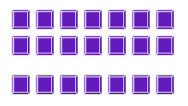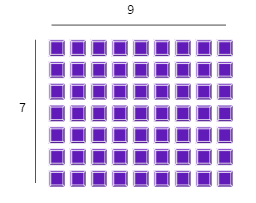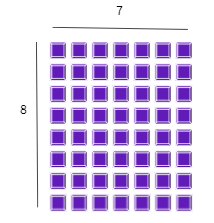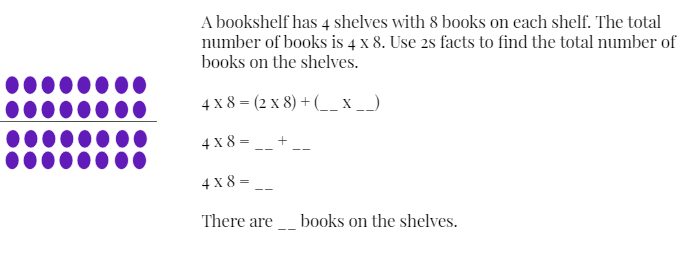Distributive Property
3 and 4 as Factors
6 and 7 as Factors
Associative Property
8 as a Factor
100

8 x 8 = (__ x 8) + (4 x 8)

8 x 8 = (4 x 8) + (4 x 8)

100

3 x 8 =___

24

100

6 x 7 = ___

42

100

What is the missing number?

8 x (3 x 6) = (8 x 3) x ___

6

100

8 x 4 = ___

32

200

5 x 7 = (3 x 7 ) + (2 x __ )

5 x 7 = (3 x 7 ) + (2 x 7)

200

7 x 3 = ___

21

200

6 x 4 = ___

24

200

What is the missing number?

5 x (2 x 9) = (5 x __) x 9

2

200

8 x 8 = ___

64

300

6 x 8 = (4 x __ ) + (2 x 8)

6 x 8 = (4 x 8) + (2 x 8)

300

Find 3 x 7

2 x 7 = __

1 x 7 = __

__ + __ =

So, 3 x 7 = __2 x 7 = 14

1 x 7 = 7

14 + 7 =

So, 3 x 7 = 21

300

You can find 7 x 9 by breaking a large array into two smaller arrays. Use smaller arrays for multiplication facts that you know, such as 2s facts, 5s facts, or doubles facts. Use two smaller arrays to find 7 x 9.5 x 9 = 45

2 x 9 = 18

45 + 18 = 63

So, 7x9 = 63

300

2 x 3 x 2

12

300

To solve a multiplication equation that has 8 as a factor, you can break it into two or more facts. You can use a 4s fact and double it to find the product. Split the 8 x 7 array into two equal groups.4 x 7 = 28

4 x 7 = 28

28 + 28 = 56

So,  8 x 7 = 56

400

Rafael broke up an array for 6 x 3 into two new arrays. Both of his new arrays are the same. What were the two arrays?

Two 3 x 3 arrays

4004 x 8 = (2 x 8) + (2 x 8)

4 x 8 = 16 + 16

4 x 8 = 32

There are 32 books on the shelves

400

Break the array into two smaller arrays using a doubles fact and solve 6x8.3 x 8 = 24

3 x 8 = 24

24 + 24 = 48

So, 6 x 8 = 48

400

5 x 2 x 4

40

4008 x 9 = (2 x 9) + (2 x 9) + (2 x 9) + (2 x 9)

8 x 9 = 18 + 18 + 18 + 18

8 x 9 = 72

500

Draw a 4 x 5 array. Break the array into 2 smaller arrays. Write and solve the multiplication facts for the smaller arrays. Then add the products to find the product of 4 x 5.

500

Draw an array to show 3 x 9. Then break apart the array to show how you can use a 2s fact and a 1s fact to solve the problem.

500

Draw a 7 x 6 array. Then, break the array into smaller arrays to find the product of 7 x 6.

500

3 x 6 x 2

36

500

Draw an array for 8 x 10. Describe how you could solve 8 x 10 by doubling a 4s fact or by using 2s facts.